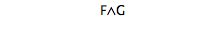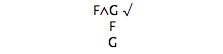# Tree Tutorial 1: Propositional Trees: Introduction

Logical System

## Tree Tutorial 1 Propositional Logic Truth Trees: Introduction

### Prerequisities

You need to know some propositional logic to be able to understand this. In particular, you need to know about the symbols used in propositional logic, truth tables, satisfiability, consistency, and semantic invalidity (by counter example). You do not need to know propositional rules of inference and derivations. [The tutorials on propositional logic elsewhere on this site give the required background. Alternatively

Colin Howson,  Logic with trees Chapter 1

would put you about where you need to be.]

### Skills to be acquired in this tutorial:

To become familiar with the notions of tree, branch, open branch, closed branch.

Colin Howson,  Logic with trees Chapter 2

[The use of trees (or 'tableaux') in logic was pioneered by Beth, Hintikka, and Smullyan, and their use was brought into a teaching context by such books as the standard Jeffrey text. See

E.W. Beth,  The Foundations of Mathematics
G.Gentzen, [1934-5] 'Untersuchungen uber das logische Schliessen', Mathematische Zeitschrift, vol 39, 1934-5, pp.176-210, 405-31
J. Hintikka  ' Two papers on Symbolic Logic', Acta Philosophica Fennica, vol 8 1955
R.C.Jeffrey,  Formal Logic: Its Scope and Limits
R.M. Smullyan,  First Order Logic

]

#### Graph theory and trees

'Trees' are a type of structure within the mathematical theory of graphs.

Graphs consist of two things: nodes (other words for these, 'vertices', 'points') and links (other words for these, 'edges', 'lines', 'ties'). In applications, nodes typically model individual people, countries, businesses, web pages, genes, amino acids, etc. Links typically model friendships, web hyperlinks, telephone lines, communications, etc. For us, nodes are going to represent formulas and the links will show the decomposition, or transformation, of a formula into other formulas.

You can think of a node and being a dot or a point, and a link as being a line or an edge which runs from a node to a node. So here is a graphThe size of a graph is the number of nodes in it. This one is of size 7.

The degree of a node is the number of links that connect to it. So, for example, the node d has degree 2 and g degree 3.

How the links are drawn is irrelevant (you can draw them as curves or straight lines or locate them differently, and the links can cross each other without that meaning anything)-- all that matters is which nodes they connect. These two graphs are actually the same graph.walk is an alternating sequence of nodes and links, which starts and ends with a node. So, in the first example graph, a->d->e is a walk. A path is a walk with no repeated nodes. The length of a walk is the number of links included in it. Two nodes are adjacent if there is a link between them.

A graph has a cycle, or is cyclic, if there is a walk of non-zero length that starts and ends on the same node (otherwise the graph is said to be acyclic).

A graph is connected if there is a path between every two nodes in it. The first example graph is not connected (because the node b cannot be reached), and the second example graph is connected.

There are a couple of different ways of explaining or defining trees (and which is preferable depends a little on what is going to be done with the resulting trees).

tree is a connected graph in which between every two nodes there is only one path (ie there are no 'cycles'). For example,is a tree (you can walk from node to node (so it is connected) but you cannot walk around in a circle (so there are no cycles)).

With a tree, there are nodes which do not have other nodes below them. These are leaves. So, in the above tree, d is the root, and c, f and b are leaves. With any leaf, there is exactly one path from the root to it, such a path is a branch.d-a-g-f is a branch in the above tree.

#### Truth Tree Rules

Trees are going to be used to 'picture' the truth conditions or requirements for a formula (then, as the technique is developed, for several formulas at once).

Any easy way to remember the rules is to regard them as having been built from the truth tables.

For example, the truth table for 'and' is

Conjunction

 F G (F∧G) True True True True False False False True False False False False

For a conjunction to be true, each of its immediate subformulas have to be true. A tree is started with the conjunction as the root, and it is extended by adding a branch containing each of the immediate subformulas. Thus

beforeafter[These images come from the running widget that we will look at shortly, the tick is just to show which formula has been extended.]

The tree extension rules are also used to grow a tree even when the formula that is being used to make the extension is not the root.

Try building some 'and' trees (click on the formula to select, choose 'Extend' from the menu).

You'll notice that when a Tree starts, the formulas it starts from are justified by 'SM'. This stands for 'Set Member' just to show that the formulas are members of the starting set of formulas.

A tree 'pictures' truth conditions. It shows what has to happen for a formula to be true. The idea is: if all the atomic formulas in a branch are true, or are assigned true, and all the negations of atomic formulas in a branch are true, ie their atomic components are assigned false, then all the formulas in the branch will be true. So, for example, (F∧G) is true if F is true and G is true, and (H∧¬I) is true if H is true and I is false. However, this is subject to a condition. Consider the formula (F∧¬F), it is not satisfiable, no assignment of truth values to its atomic components can make it true. If you do a tree for a formula like this (Tree 3 above), you will end up with a branch that has both F and ¬F in it; this means that you will be trying to assign F both true and false (which is impossible).

Any branch that contains a formula and the negation of that formula is said to be closed. (And any branch that is not closed is open.)

The running software allows you to close (suitable) branches. To do this you have to select two formulas, one of the formulas has to be the negation of the other, then you click the 'Close' button. To select (or unselect) a formula, click on it if you have a mouse, or touch it with your finger, if you are using a touchscreen device

## Exercises to accompany Tutorial 1

### Exercise 1 (of 1) (very brief):

For Tree 3 above, extend the tree, then select both F and ¬F (click on the first, click on the second) then select 'Close' from the menu. This is just to introduce you to the notation for closed branches.